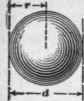## Solids And Curved Surfaces

C = convex surface; S = whole surface;

= C + area of end or ends; A = area larger base or end; a = area smaller base or end; P = perimeter of larger base; p = perimeter of smaller base; D = larger diameter; d = smaller diameter; V = volume of solid. In a cylinder or prism,

A = a, P = p, and D = d.## Cylinder

C = Ph = 3.1416dh; S = 3.1416dh + 1.5708d2; V = Ah = .7854d2A.

## Frustum Of Cylinder

h = 1/2 sum of greatest and least heights;

C = Ph = 3.1416 d h;

S = 3.1416 d h + .7854 d2 + area of elliptical top; V = A h = .7854 d2 h.## Prism Or Parallelopiped

C = Ph; S=Ph+2A; V = Ah. For prisms with regular polygon as bases, P = length of one side X number of sides.

To obtain area of base, if it is a polygon, divide it into triangles, and find sum of partial areas.## Frustum Of Prism

If a section perpendicular to the edges is a triangle, square, parallelogram, or regular polygon, sum of lengths of edges , . . .

V = X area of right sec-number of edges tion.## Cone

C = 1/2 PI = l.5708dl: S = 1.5708 dl + .7854d2;

V = Ah/3 = .2618d2h.

## Frustum Of Cone

C = 1/2 l(P + p) = 1.5708l(D + d); S = 1.5708l(D + d)+.7854 (D2 + d2); V =.2618h(D2 + Dd + d2).## Pyramid

C =1/2Pl:

S = 1/2Pl + A;

V=Ah/3

To obtain area of base, divide it into triangles, and find their sum.

## Frustum Of Pyramid

C = 1/2l(P + p);

S = 1/2(P +p)+A + a;## Sphere

S = 3.1416 d2 = 12.5664r2; V = .5236d3 = 4.1888 r3.

## Circular Ring

D = mean diameter; R = mean radius.

S = 4╥2Rr = 9.8696Dd.

V = 2╥2Rr2 = 2.4674Dd2.## Prismoid

A prismoid is a solid having two parallel plane ends, the edges of which are connected by plane triangular or quadrilateral surfaces. A = area one end; a = area of other end; m = area of section mid way between ends;

I = perpendicular distance between ends;

V = 1/6l(A + a + 4m).

The area to is not in general a mean between the areas of the two ends, but its sides are means between the corresponding lengths of the ends.

Approximately, V =[(A+a)/2] l.